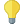#Apply Forecast (Time Series)

## Synopsis

This operator uses a forecast model to predict the next values of a time series.

## Description

The type of forecast depends on the provided model, which also includes the time series data. This is a difference to the Apply Model operator, which applies a model on an ExampleSet. The length of the forecast is an user defined parameter.

## Differentiation

###Apply Model

Apply model can also used to predict new values. This is normally the case for models created by predictive algorithms (i.e., classification or regression models). But in contrast to the Apply Forecast operator the Apply Model operator requires an ExampleSet that is compatible with the model. Because a time series forecast model is highly dependend on the data it was fitted to, this data is already included in the forecast model.

## Input

•forecast model (IOObject)

The forecast model for which new values will be generated. It also contains the values of the original time series the model was trained on.

## Output

•example set (Data Table)

ExampleSet containing the forecasted time series values. Depending on the selected parameters, the original values are also included.

•original (IOObject)

The model that was given as input is passed through without changes.

## Parameters

• forecast_horizon

This parameter specifies the length of the forecast. It states how many new points are generated by the model. A long forecast leads to a high uncertainty because fewer information is available.

Range:

If this parameter is set to true an additional attribute containing the original values of the time series is added to the result. The forecast attribute will have missing values for the original entries and the new attribute will have missing for the forecasted entries.

Range: